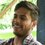# Open Challenge :)

Let $x,y\quad \in \quad R$ such that $\cos { x } \cos { y } \quad +\quad \quad 2\sin { y } \quad +\quad 2\sin { x } \cos { y } \quad =\quad 3$.
Then find the value of : $\tan ^{ 2 }{ x } +\quad 5\tan ^{ 2 }{ y } \quad =\quad ?$.

It is really very beautiful question. That's why I share this with our Brilliant community.

Use any Tool of mathematics. There are no restrictions

(You may use Vectors, Complex numbes, Trigonometry, etc .)Note by Deepanshu Gupta
6 years, 8 months ago

This discussion board is a place to discuss our Daily Challenges and the math and science related to those challenges. Explanations are more than just a solution — they should explain the steps and thinking strategies that you used to obtain the solution. Comments should further the discussion of math and science.

When posting on Brilliant:

• Use the emojis to react to an explanation, whether you're congratulating a job well done , or just really confused .
• Ask specific questions about the challenge or the steps in somebody's explanation. Well-posed questions can add a lot to the discussion, but posting "I don't understand!" doesn't help anyone.
• Try to contribute something new to the discussion, whether it is an extension, generalization or other idea related to the challenge.

MarkdownAppears as
*italics* or _italics_ italics
**bold** or __bold__ bold
- bulleted- list
• bulleted
• list
1. numbered2. list
1. numbered
2. list
Note: you must add a full line of space before and after lists for them to show up correctly
paragraph 1paragraph 2

paragraph 1

paragraph 2

[example link](https://brilliant.org)example link
> This is a quote
This is a quote
    # I indented these lines
# 4 spaces, and now they show
# up as a code block.

print "hello world"
# I indented these lines
# 4 spaces, and now they show
# up as a code block.

print "hello world"
MathAppears as
Remember to wrap math in $$ ... $$ or $ ... $ to ensure proper formatting.
2 \times 3 $2 \times 3$
2^{34} $2^{34}$
a_{i-1} $a_{i-1}$
\frac{2}{3} $\frac{2}{3}$
\sqrt{2} $\sqrt{2}$
\sum_{i=1}^3 $\sum_{i=1}^3$
\sin \theta $\sin \theta$
\boxed{123} $\boxed{123}$

Sort by:

Hint: Write the given equation as

$\bigg(\cos x+2\sin x\bigg)\cos y+2\sin y=3$

$\ddot\smile$

- 6 years, 8 months ago

@Karthik Kannan Did you mean this way :

$E\quad \quad \quad =\quad (\cos x+2\sin x)\cos y+2\sin y\\ \\ { E }_{ max }\quad =\quad \sqrt { { (\cos x+2\sin x) }^{ 2 }+\quad { (2) }^{ 2 } } \quad \\ \\ \quad \quad \quad \quad =\quad \sqrt { \cfrac { 13 }{ 2 } \quad -\quad \cfrac { 3 }{ 2 } \cos { 2x } \quad +\quad 2\sin { 2x } } \\ \quad \quad \quad \quad \\ \quad { E }_{ max }\quad \in \quad \left[ 2\quad ,\quad 3 \right] \\ \\ { E }_{ max }\quad \le \quad 3\\ \\ But\quad \quad \quad { E }_{ max }\quad =\quad 3\\$.

Now using boundedness of function ! Great ! This is also Nice Method !!

- 6 years, 8 months ago

if x,y belongs to R , then the first expression should be true for all real values of x, but we can see here its true only for some specific

- 6 years, 8 months ago

- 6 years, 8 months ago

wait be clear , I said : $x,y\quad \in \quad R$. which means x and y are real numbers. it dosn't mean that that expression is true for every x,y .

And if i say $\forall \quad x,y\quad \in \quad R\quad \quad$. then it means this expression is true for every x ( which means it is identity )

- 6 years, 8 months ago

HINT: USE cauchy inequality

- 6 years, 8 months ago

Yes that is best!!

- 6 years, 8 months ago

- 6 years, 8 months ago

Let two Vectors such that :

Now Verify that :

$\overset { \rightarrow }{ A } .\overset { \rightarrow }{ B } \quad =\quad \left| \overset { \rightarrow }{ A } \right| \left| \overset { \rightarrow }{ B } \right|$.

So angle between these two vectors is zero degree , means they are parallel ,

Now use simple condition of Parallel Vectors and get the Answer ! :)

@Aman Gautam I Hope you Got it !

- 6 years, 8 months ago